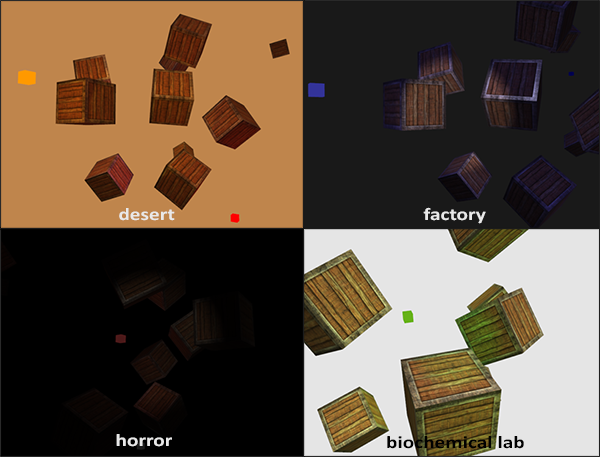# 多光源

GLSL中的函数与C语言的非常相似，它需要一个函数名、一个返回值类型。并且在调用前必须提前声明。接下来我们将为下面的每一种光照来写一个函数。

out vec4 color;

void main()
{
// 定义输出颜色
vec3 output;
// 将平行光的运算结果颜色添加到输出颜色
output += someFunctionToCalculateDirectionalLight();
// 同样，将定点光的运算结果颜色添加到输出颜色
for(int i = 0; i < nr_of_point_lights; i++)
output += someFunctionToCalculatePointLight();
// 添加其他光源的计算结果颜色（如投射光）
output += someFunctionToCalculateSpotLight();

color = vec4(output, 1.0);
}


## 平行光

struct DirLight {
vec3 direction;

vec3 ambient;
vec3 diffuse;
vec3 specular;
};
uniform DirLight dirLight;


vec3 CalcDirLight(DirLight light, vec3 normal, vec3 viewDir);


Important

vec3 CalcDirLight(DirLight light, vec3 normal, vec3 viewDir)
{
vec3 lightDir = normalize(-light.direction);
// 计算漫反射强度
float diff = max(dot(normal, lightDir), 0.0);
// 计算镜面反射强度
vec3 reflectDir = reflect(-lightDir, normal);
float spec = pow(max(dot(viewDir, reflectDir), 0.0), material.shininess);
// 合并各个光照分量
vec3 ambient  = light.ambient  * vec3(texture(material.diffuse, TexCoords));
vec3 diffuse  = light.diffuse  * diff * vec3(texture(material.diffuse, TexCoords));
vec3 specular = light.specular * spec * vec3(texture(material.specular, TexCoords));
return (ambient + diffuse + specular);
}


## 点光源

struct PointLight {
vec3 position;

float constant;
float linear;

vec3 ambient;
vec3 diffuse;
vec3 specular;
};
#define NR_POINT_LIGHTS 4
uniform PointLight pointLights[NR_POINT_LIGHTS];


Important

vec3 CalcPointLight(PointLight light, vec3 normal, vec3 fragPos, vec3 viewDir);


// 计算定点光在确定位置的光照颜色
vec3 CalcPointLight(PointLight light, vec3 normal, vec3 fragPos, vec3 viewDir)
{
vec3 lightDir = normalize(light.position - fragPos);
// 计算漫反射强度
float diff = max(dot(normal, lightDir), 0.0);
// 计算镜面反射
vec3 reflectDir = reflect(-lightDir, normal);
float spec = pow(max(dot(viewDir, reflectDir), 0.0), material.shininess);
// 计算衰减
float distance    = length(light.position - fragPos);
float attenuation = 1.0f / (light.constant + light.linear * distance +
// 将各个分量合并
vec3 ambient  = light.ambient  * vec3(texture(material.diffuse, TexCoords));
vec3 diffuse  = light.diffuse  * diff * vec3(texture(material.diffuse, TexCoords));
vec3 specular = light.specular * spec * vec3(texture(material.specular, TexCoords));
ambient  *= attenuation;
diffuse  *= attenuation;
specular *= attenuation;
return (ambient + diffuse + specular);
}


## 把它们放到一起

void main()
{
// 一些属性
vec3 norm = normalize(Normal);
vec3 viewDir = normalize(viewPos - FragPos);

// 第一步，计算平行光照
vec3 result = CalcDirLight(dirLight, norm, viewDir);
// 第二步，计算顶点光照
for(int i = 0; i < NR_POINT_LIGHTS; i++)
result += CalcPointLight(pointLights[i], norm, FragPos, viewDir);
// 第三部，计算 Spot light
//result += CalcSpotLight(spotLight, norm, FragPos, viewDir);

color = vec4(result, 1.0);
}


glUniform1f(glGetUniformLocation(lightingShader.Program, "pointLights.constant"), 1.0f);


glm::vec3 pointLightPositions[] = {
glm::vec3( 0.7f,  0.2f,  2.0f),
glm::vec3( 2.3f, -3.3f, -4.0f),
glm::vec3(-4.0f,  2.0f, -12.0f),
glm::vec3( 0.0f,  0.0f, -3.0f)
};## 练习

• 创建一个表示手电筒光的结构体Spotlight并实现CalcSpotLight(…)函数：解决方案
• 你能通过调节不同的光照属性来重新创建一个不同的氛围吗？解决方案